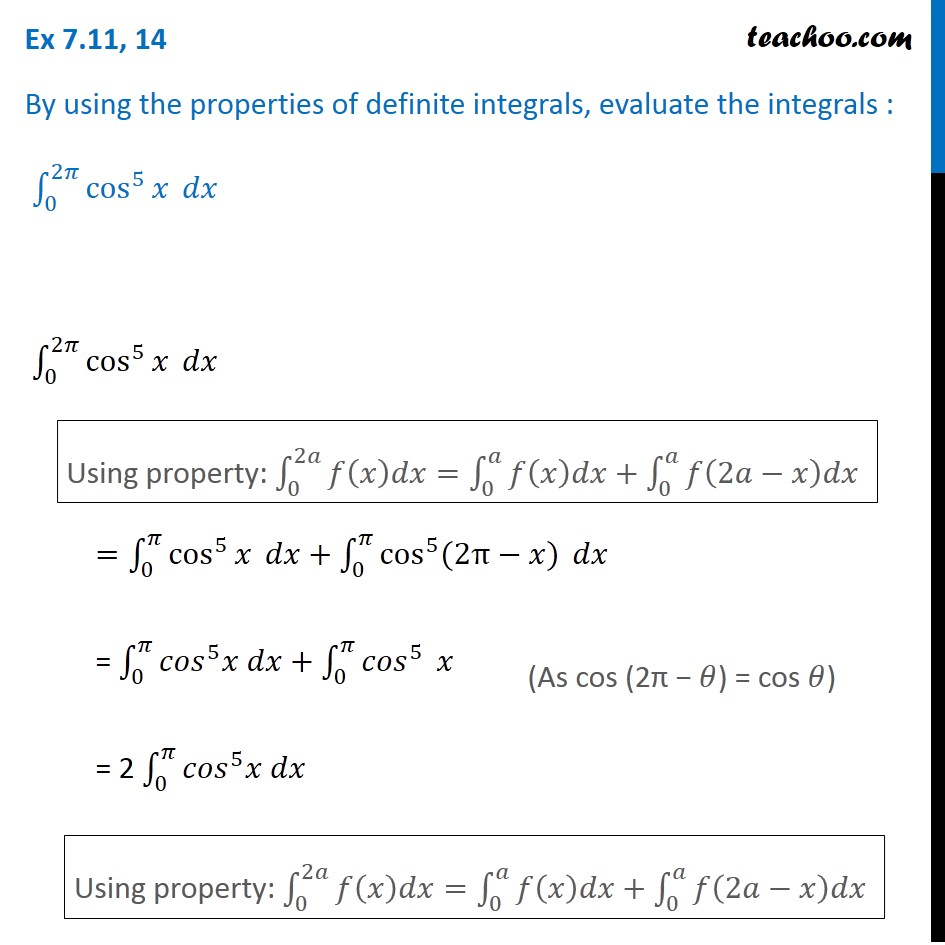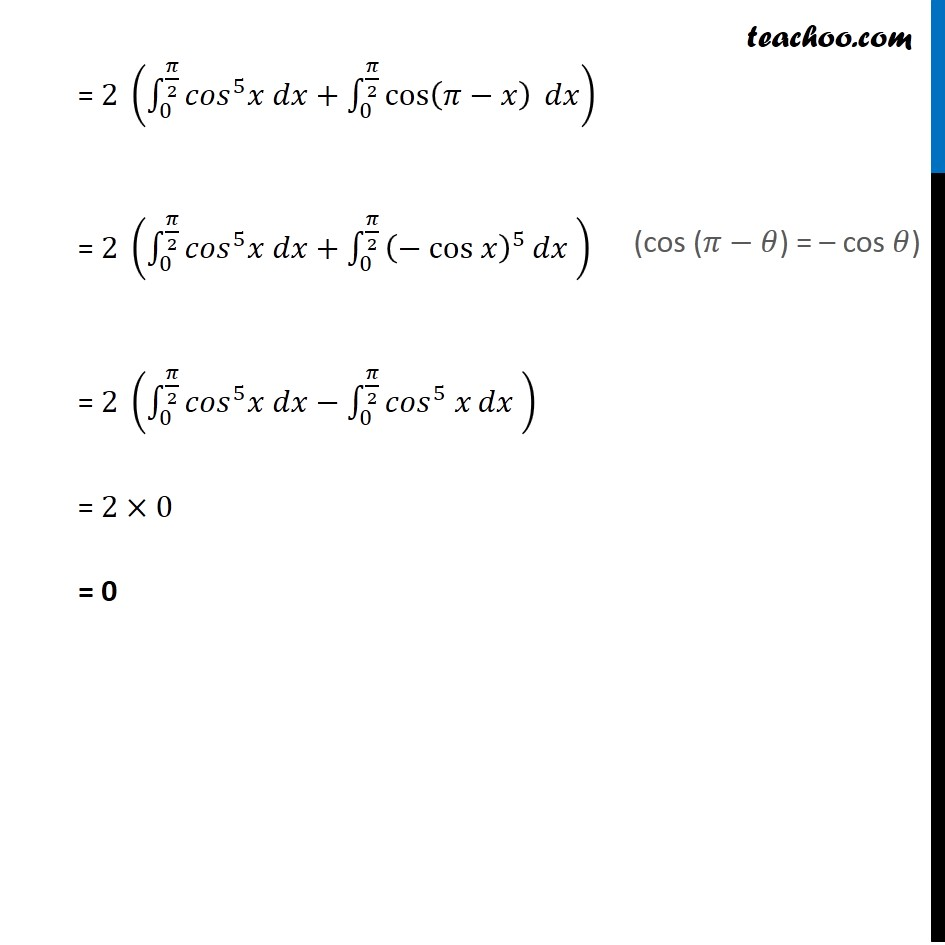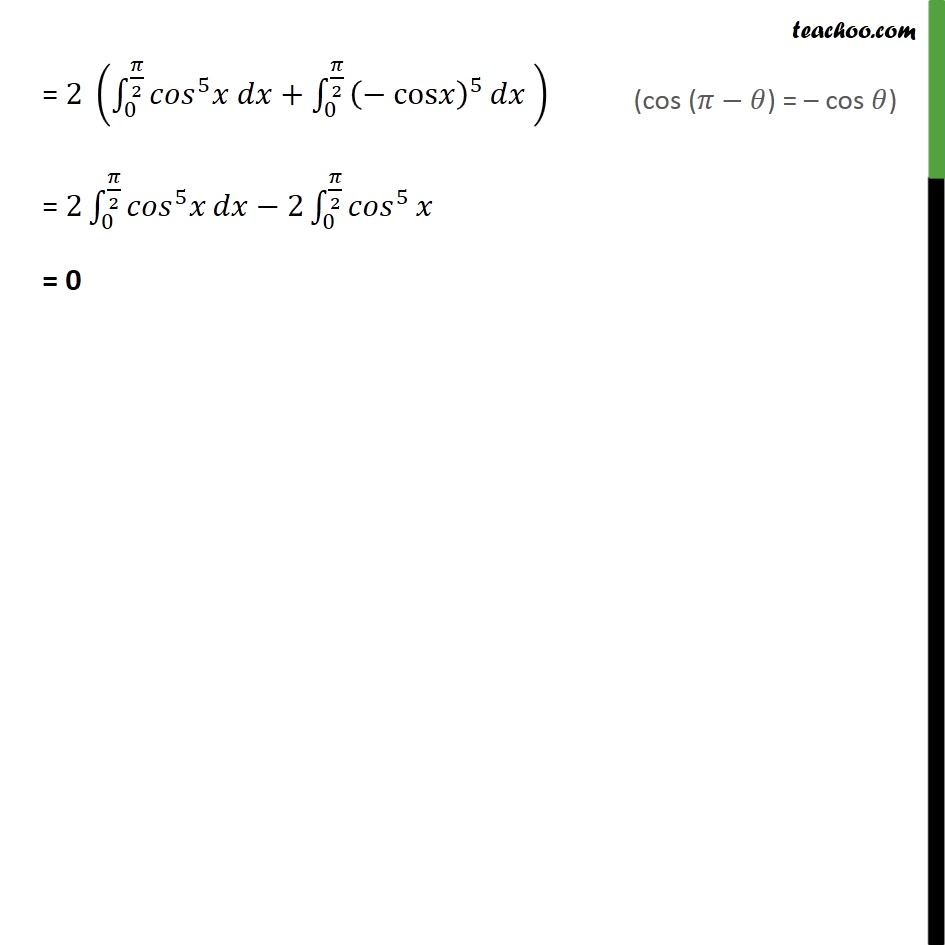Definite Integration by properties - P6

Chapter 7 Class 12 Integrals
Concept wiseLearn in your speed, with individual attention - Teachoo Maths 1-on-1 Class

### Transcript

Ex 7.10, 14 By using the properties of definite integrals, evaluate the integrals : ∫_0^2𝜋▒cos^5⁡𝑥 𝑑𝑥 ∫_0^2𝜋▒cos^5⁡𝑥 𝑑𝑥 =∫_0^𝜋▒cos^5⁡𝑥 𝑑𝑥+∫_0^𝜋▒〖cos^5 (2π−𝑥)〗 𝑑𝑥 = ∫_0^𝜋▒〖〖𝑐𝑜𝑠〗^5 𝑥 𝑑𝑥+∫_0^𝜋▒〖𝑐𝑜𝑠〗^5 〗 𝑥 = 2 ∫_0^𝜋▒〖〖𝑐𝑜𝑠〗^5 𝑥 𝑑𝑥〗 Using property: ∫_0^2𝑎▒〖𝑓(𝑥)𝑑𝑥=∫_0^𝑎▒〖𝑓(𝑥)𝑑𝑥+∫_0^𝑎▒𝑓(2𝑎−𝑥)𝑑𝑥〗〗 (As cos (2π − 𝜃) = cos 𝜃) Using property: ∫_0^2𝑎▒〖𝑓(𝑥)𝑑𝑥=∫_0^𝑎▒〖𝑓(𝑥)𝑑𝑥+∫_0^𝑎▒𝑓(2𝑎−𝑥)𝑑𝑥〗〗 = 2 (∫_0^(𝜋/2)▒〖〖𝑐𝑜𝑠〗^5 𝑥 𝑑𝑥+∫_0^(𝜋/2)▒〖cos⁡(𝜋−𝑥) 〗〗 𝑑𝑥) = 2 (∫_0^(𝜋/2)▒〖〖𝑐𝑜𝑠〗^5 𝑥 𝑑𝑥+∫_0^(𝜋/2)▒〖(− cos 𝑥)^5⁡𝑑𝑥 〗〗) = 2 (∫_0^(𝜋/2)▒〖〖𝑐𝑜𝑠〗^5 𝑥 𝑑𝑥−∫_0^(𝜋/2)▒〖〖〖𝑐𝑜𝑠〗^5 𝑥〗⁡𝑑𝑥 〗〗) = 2×0 = 0 (cos (𝜋−𝜃) = – cos 𝜃)